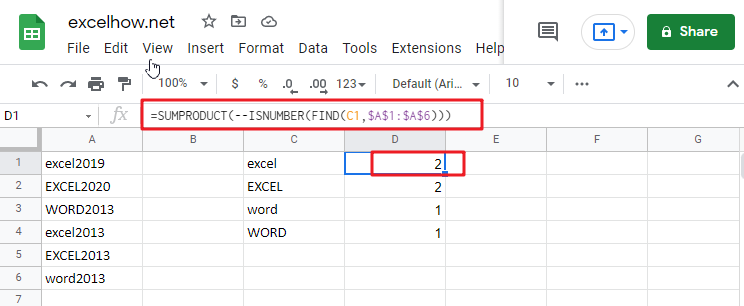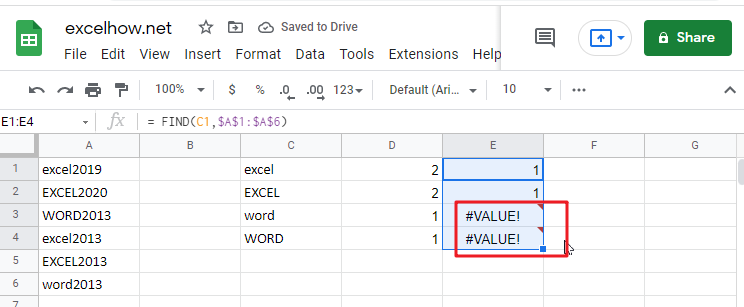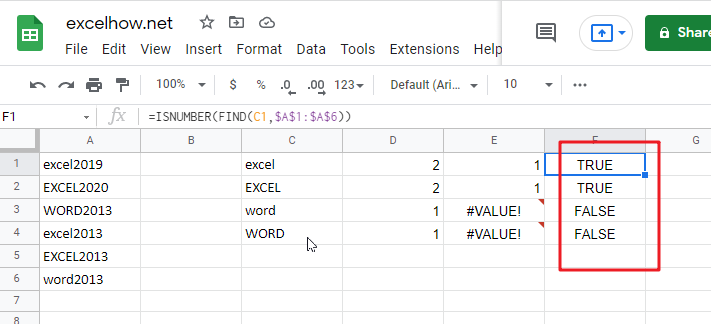# Count Cells that are Case Sensitive in Google SheetsSimple generic formula:

`=SUMPRODUCT(--ISNUMBER(FIND(value, range)))`

## Summary

You may use a formula depending on the ISNUMBER and FIND functions and the SUMPRODUCT function to calculate the number of cells that contain specified text (i.e., hold a substring), taking into consideration upper and lower case. D1 in the example displayed has the following formula, which has been written down:

`=SUMPRODUCT(--ISNUMBER(FIND(C1,\$A\$1:\$A\$6)))`

## Let’s See How This Formula Works

In this example, the purpose is to count the number of times each code appears as a substring in a case-sensitive manner, as shown below. When calculating text values, the functions COUNTIF and COUNTIFS are excellent choices. However, since these functions are not case-sensitive, they cannot be utilized to address this issue. To check for substrings, the FIND function should be used in conjunction with the ISNUMBER function, and the SUMPRODUCT function should be used to sum up, the results.

`= FIND(C1,\$A\$1:\$A\$6)`

The FIND function is case-sensitive and accepts three parameters: find text, within the text, and start num. It is also case-sensitive and takes three arguments. Here is the text we are searching for, and this is the text that we are searching inside. Start num is the number of characters to begin searching in find text. Because start num defaults to 1, we are not supplying a number in this instance. This is necessary since we always want FIND to begin with the first character. When finding the text is discovered inside within text, FIND returns the position of the found text as a number: When finding the text is located within text, FIND returns the position of the found text as a number:Note: When the find text function fails to yield a result, FIND returns the #VALUE! Error.

`=ISNUMBER(FIND(C1,\$A\$1:\$A\$6))`

This implies that we may be using the ISNUMBER function to transform the output of the FIND function into a TRUE or FALSE value using the ISNUMBER function. A TRUE value will arise from any number, while a FALSE value will result from any error:As demonstrated in the example, we have four substrings in column C and a variety of codes in columns A1:A6, representing the data for the designated range. Specifically, we want to know how many times each substring in C1:C4 occurs in A1:A6, and we want to know how many times each substring is case-sensitive.

### Related Functions

• Excel ISNUMBER function
The Excel ISNUMBER function returns TRUE if the value in a cell is a numeric value, otherwise it will return FALSE.The syntax of the ISNUMBER function is as below:= ISNUMBER (value)…